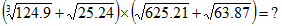Welcome to your Quantitative Aptitude Approximation Quiz -1

Calculate the Approximate Value:
25.21 − 212.02 ÷ 38.87= 8 × ?Deselect Answer

Calculate the Approximate Value :